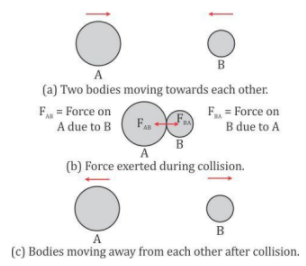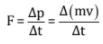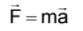# ICSE Revision Notes for Force Class 10 Physics

 Chapter Name Force Topics Covered ForceNewton's Laws of MotionTranslational and Rotational MotionTorqueCircular Motion Related Study

## Force

Kinds of Forces

• Force is a physical cause which changes (or tends to change) either the size or shape or the state of rest or motion of the body.
• The forces which act on bodies when they are in physical contact are called contact forces.
• When a body moves over a rough surface, a force acts on the body in a direction opposite to the motion of the body along the surface of contact. This is called the frictional force or the force of friction.
• When a person moves towards the right on a road, the force of friction acts on him towards the left. This force resists his motion on the road.
• When a body is placed on a surface, the body exerts a force equal to its weight in the downward direction on the surface. However, the body does not move (or fall) because the surface exerts an equal and opposite force on it, which is called the normal reaction force.
• When a body is suspended by a string, the body pulls the string vertically downwards due to its weight. In its stretched condition, the string pulls the body upwards by a force which balances the weight of the body. This force developed in the string is called the tension force T.• The spring has a tendency to return to its original form. Similarly, when one end of a spring is kept fixed, the spring is found to exert a force at its other end which is directly proportional to the displacement, and the force exerted is in a direction opposite to the direction of displacement. This force is called the restoring force.
• When two bodies collide, they push each other. As a result, equal and opposite forces act on each body.• The forces experienced by bodies even without being physically touched are called non-contact forces or forces at a distance.
• In the Universe, each particle attracts another particle because of its mass. This force of attraction between the particles is called the gravitational force.
• The force on a body due to the Earth’s attraction is called the force of gravity. It causes the movement of the body towards the Earth, i.e. downwards, if the body is free to move. The body also attracts the Earth by an equal amount of force, but no motion is caused in the Earth because of its huge mass.
• Two like charges repel, while two unlike charges attract each other. The force between the charges is called the electrostatic force.
• Two like magnetic poles repel each other, while two unlike magnetic poles attract each other. The force between the magnetic poles is called the magnetic force.

General Character of Non-contact Forces

1. The gravitational force is always of an attractive nature, while the electrostatic force and the magnetic force can be either attractive or repulsive.
2. The magnitude of non-contact forces on the two bodies varies inversely as the square of the distance of separation between them. It decreases with an increase in separation and increases as the separation decreases.

### Newton's laws of Motion

1. Newton’s First Law of motion

• Newton’s First Law: A body remains in the state of rest or of uniform motion in a straight line unless an external force is applied on it.
• The property of an object by virtue of which it neither changes its state nor tends to change its state is called inertia. It is an inherent property of each object.

2. Newton’s Second Law of Motion

• Newton’s Second Law: The rate of change of momentum of a body is directly proportional to the applied force and takes place in the direction in which the force acts.Momentum

• The force required to stop a moving body is directly proportional to the mass and velocity of the body.
• The momentum ‘p’ of a body is defined as the product of mass ‘m’ and velocity ‘v’ of the body.
p = mv
• If a body is at rest, then its momentum will be zero.
• Momentum has both magnitude as well as direction; hence, it is a vector quantity.
• The SI unit of momentum is kg m/s
• Rate of change of momentum = (Change in momentum)/Time = m(v - u)/t = ma
• Thus, the rate of change of momentum is the product of mass and acceleration.
• From Newton’s law,
F ∝ ma or F = kma
• In SI units, k = 1,
F = ma
• In the vector form, the above equation is written as,• The acceleration produced in a body of a given mass is directly proportional to the force applied on it.
a ∝ F
• The acceleration produced in a body is inversely proportional to the mass of the body.
1/a ∝ 1/m

3. Newton’s Third Law of Motion

To every action, there is an equal and opposite reaction.

• When you pull a spring with both hands, you feel an equal force trying to pull the spring back.• Thus, in an interaction of two bodies, there are action and reaction forces present. However, we should note that the action and reaction forces act on two different bodies.
• In the CGS system, the unit of force is dyne.
1 dyne = 1 g × 1 cm s−2
• SI unit: The SI unit of force is newton (N).
1 N = 1 kg × 1 m s−2
• One newton (N) is that force which when acting on a body of mass 1 kg produces an acceleration of 1 m s−2in it.
1 N = 105dyne
• The gravitational unit of force in the MKS system is kilogram force (kgf).
1 kgf = 1 kg × 9.8 m s−2
1 kgf = 9.8 N
• One kilogram force is the force with which the Earth pulls a body of mass 1 kg towards itself.
• The three equations of motion considered for a body moving with uniform acceleration are:
1. v = u + at
2. s = ut + 1/2 at2
3. v2 = u2 + 2as
Here, ‘u’ is the initial velocity, ‘v’ is the final velocity, ‘a’ is the acceleration and ‘s’ is the distance.

### Translational and Rotational Motion

• When a force acts on a stationary rigid body which is free to move, the body starts moving in a straight path in the direction of the force. This is called linear or translational motion.
• When the body is pivoted at a point, the force applied on the body at a suitable point rotates the body about the axis passing through the pivoted point. This is called rotational motion.

Moment of a Force or Torque

• The turning effect of the force acting on the body about an axis is due to the moment of force or torque.
• The factors affecting the turning of a body are:
I. Magnitude of the force applied
II. Distance of the line of action of the force from the axis of rotation
• The moment of force (or torque) is equal to the product of the magnitude of the force and the perpendicular distance of the line of action of force from the axis of rotation.

Clockwise and Anticlockwise Moments

• The direction of turning (or sense of rotation) produced on the body depends on the point of application of the force and on the direction of the force.
• Conventionally, when the turning effect on the body is anticlockwise, the moment of force is called the anticlockwise moment and it is taken as positive, while if the turning effect on the body is clockwise, the moment of force is called the clockwise moment and it is taken as negative.
• The SI unit of the moment of force is newton metre (N m).
• In gravitational units, the MKS unit is (kgf m) and the CGS unit is (gf m).
1 N m = 107dyne cm
1 kgf m = 9.8 N m
1 gf m 980 dyne cm
• For turning a steering wheel, a force is applied tangentially on the rim of the wheel. The sense of rotation of the wheel is changed by changing the point of application of force without changing the direction of force.• The turning of a body about an axis depends not only on the magnitude of the force but also on the perpendicular distance of the line of action of the applied force from the axis of rotation.
• A single force applied on a pivoted body alone does not cause turning or rotation of the body.
• The turning is always produced by a pair of forces.
• Such a pair of forces is called a couple. Thus, two equal and opposite parallel forces not acting along the same line form a couple. A couple is always needed to produce rotation.
• For example, when we open a door, the rotation of the door is produced by a couple consisting of two forces:
(1) the force which we exert at the handle of the door, and
(2) an equal and opposite force of reaction at the hinge.Action of a Couple

The perpendicular distance between the two forces is called the couple arm.

• The two forces cannot produce translational motion as their resultant sum is zero. However, each force has the turning effect on the bar in the same direction, so the two forces together form a couple which rotates the bar about the point O.

Equilibrium of Bodies

• When several forces acting on a body produce no change in its state of rest or of motion, the body is said to be in equilibrium.
• When a body remains in the state of rest under the influence of the applied forces, the body is in static equilibrium.
• When a body remains in the same state of motion under the influence of the applied forces, the body is said to be in dynamic equilibrium.

Conditions for Equilibrium

1. The resultant of all the forces acting on the body should be equal to zero.
2. The resultant moment of all the forces acting on the body about the point of rotation should be zero, i.e. the sum of the anticlockwise moments about the axis of rotation must be equal to the sum of the clockwise moments about the same axis.

Principle of Moments

• If the algebraic sum of moments of all the forces acting on the body about the axis of rotation is zero, then the body is in equilibrium. This is called the principle of moments.
Sum of anticlockwise moments Sum of clock = wise moments

### Centre of Gravity

Centre of Gravity and balance point

• When a body is freely suspended from a point, it comes to rest (or in balance) in such a position that its centre of gravity lies vertically below the point of suspension.
• It is possible because the algebraic sum of moments of the weights of all the particles about the point of suspension is zero.

### Uniform Circular Motion

Centripetal and Centrifugal Force

• The force needed to make an object travel in a circular path is known as centripetal force. ∙ This force is always directed towards the centre of the circle at each point of its path. Hence, for a body moving uniformly in a circular path, there must be a force to provide the centripetal force required for circular motion.
• In an atom, an electron moves around the nucleus in a circular path for which the centripetal force is obtained from the electrostatic force of attraction on the electron by the nucleus.
• A planet moves around the Sun in a nearly circular path for which the gravitational force of attraction on the planet by the Sun provides the necessary centripetal force.
• The force acting on a body away from the centre of the circular path is called the centrifugal force.
• It is not a real force. However, it is considered to describe a certain motion and is called fictitious force or virtual force.
• Its magnitude is the same as centripetal force. It is however not a reaction force of centripetal force, because both the forces act on the same body performing circular motion.
• A bucket filled with water is swirled around. The water does not spill out of it because of the centrifugal force acting on it away from the centre.